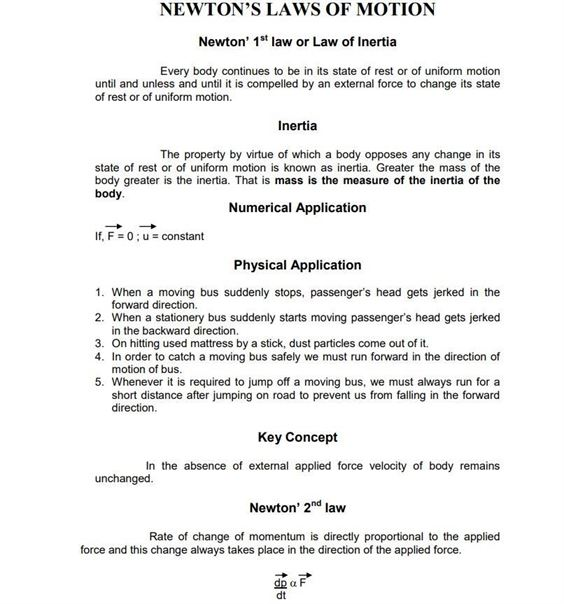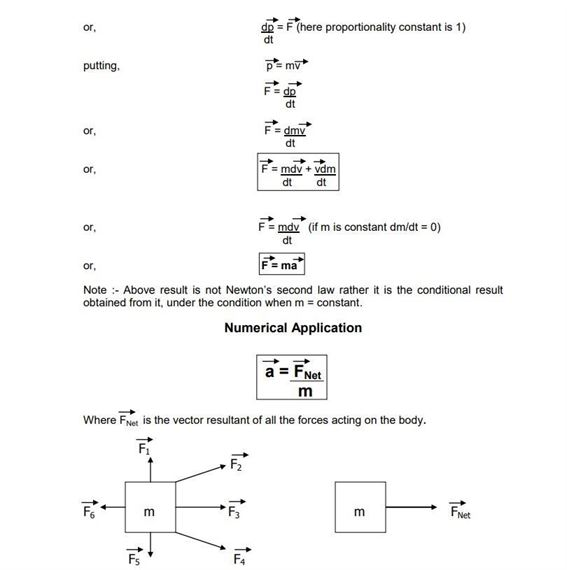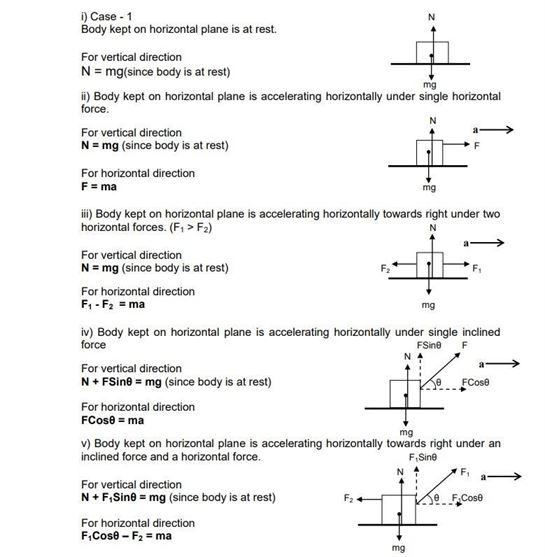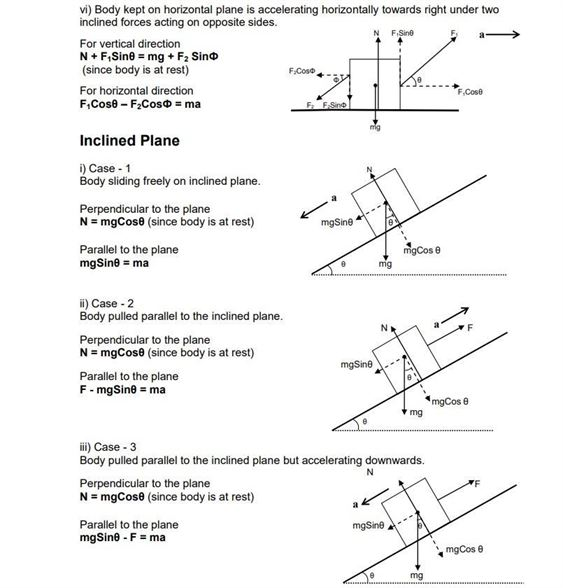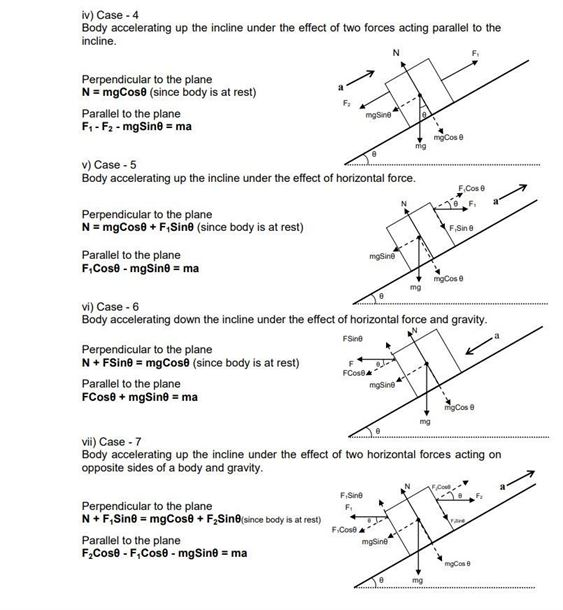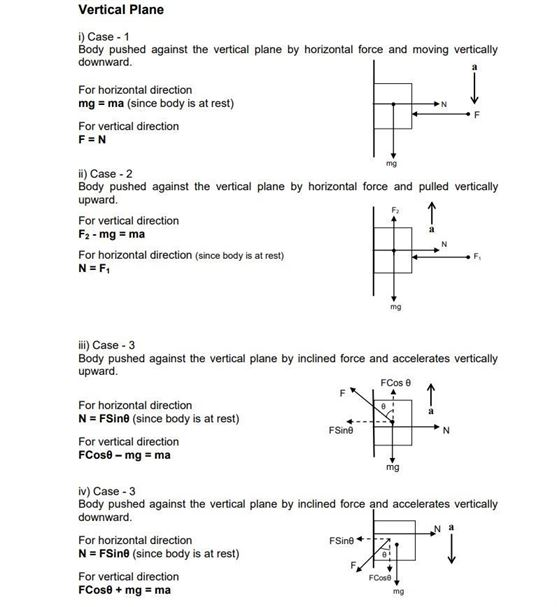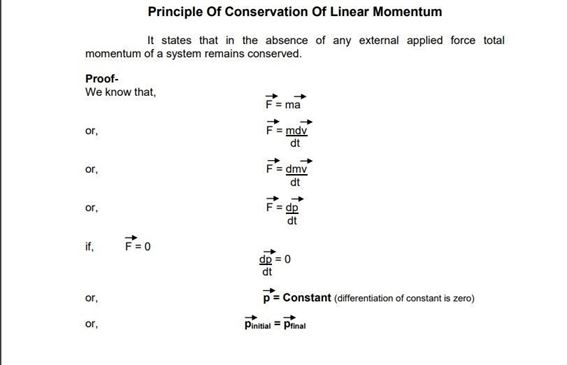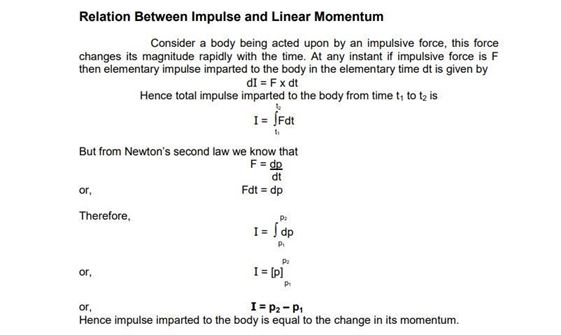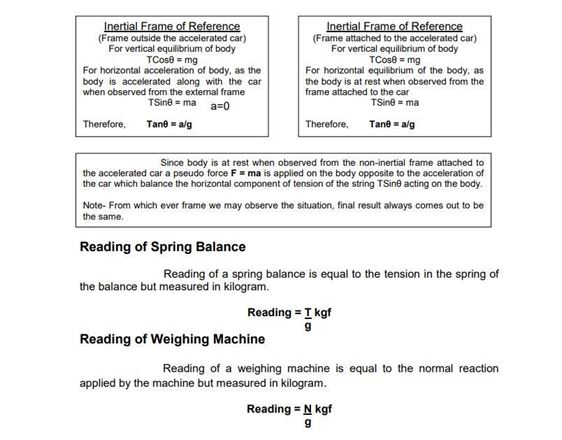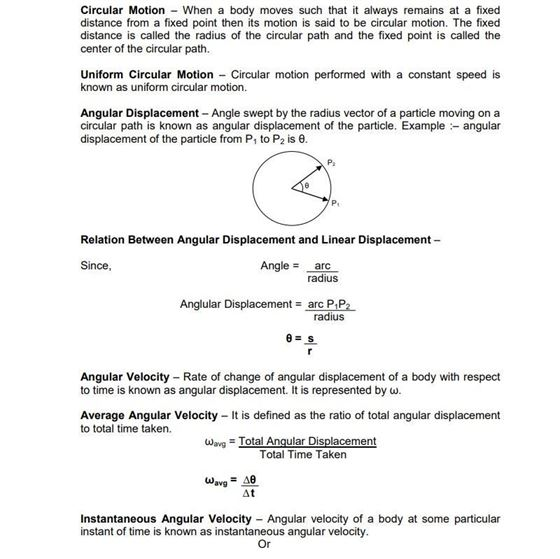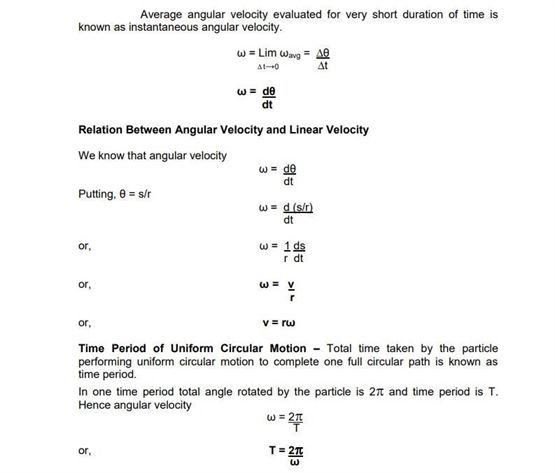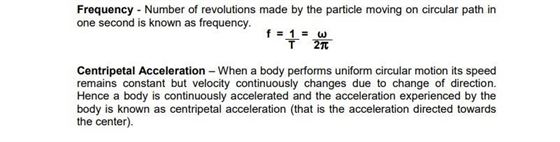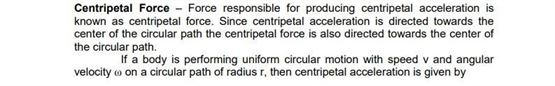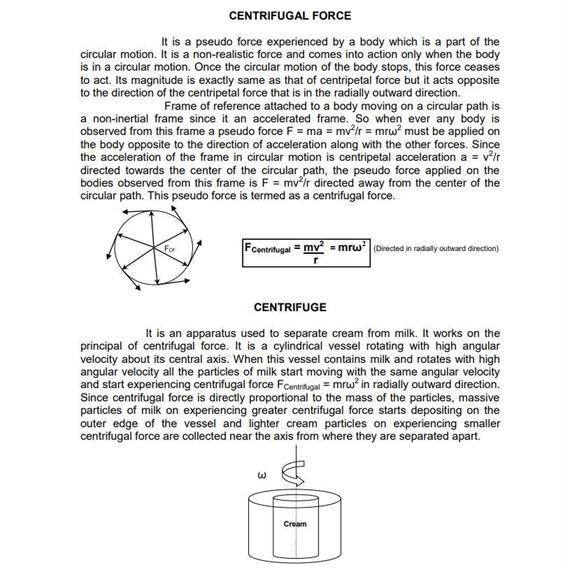# Physics Revision Notes For Class 11 Chapter 5 Laws of Motion

Class 11 physics revision notes chapter 5 laws of motion is very important if students are preparing for exams and need a reference material by their side. They will be made aware of all the newtons laws of motion and their application along with formulas. We are aware of the three laws in Physics that are:

• Newton’s first law states that every object will remain at rest or in uniform motion in a straight line unless compelled to change its state by the action of an external force.
• The second law explains how the velocity of an object changes when it is subjected to an external force. The law defines a force to be equal to change in momentum (mass times velocity) per change in time
• The third law states that for every action (force) in nature there is an equal and opposite reaction

These laws if understood thoroughly with the help of these notes will prove to be helpful when it comes to solving practical and complex problems in later stages of academics. They will get to know about centripetal and centrifugal force along with their applications with examples.

You can get here the physics revision notes for class 11 chapter 5 laws of motion here.